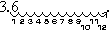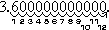Standard

# UNIT 1: REAL NUMBERS

Page

## Click here to go to the IXL website for all kinds of 8th grade topics and review problems.

To practice Classifying Real Numbers click here.

# REAL NUMBERS

In this unit we went over Real Numbers and the classification system that is set up with Real Numbers. Here is the Rational Numbers Poster we created in classWe used Nesting boxes to demonstrate how the sub groups are inside each other.

 Description of Each Set of Numbers Natural The natural numbers (also known as counting numbers) are the numbers that we use to count. It starts with 1, followed by 2, then 3, and so on.1, 2, 3, 4… Whole The whole numbers are a slight “upgrade” of the natural numbers because we simply add the number zero to the current set of natural numbers. Think of whole numbers as natural numbers and the number zero.0, 1, 2, 3, 4… **Remember a hole in the ground is shaped like the number zero!** Integers The integers include all whole numbers together with the “opposites” of the natural numbers (their negatives).… -4, -3, -2, -1, 0, 1, 2, 3, 4 … Rational The rational numbers are numbers which can be expressed as ratio of integers. That means, if we can write a given number as a fraction where the numerator and denominator are both integers; then it is a rational number.Caution: The denominator cannot equal to zero. Rational numbers can also appear in decimal form. If the decimal number either terminates or repeats, then it is possible to write it as a fraction with an integer numerator and denominator. Thus, it is rational as well Irrational The irrational numbers are all numbers which when written in decimal form does not repeat and does not terminate. Real The real numbers includes both the rational and irrational numbers. Remember that under the rational number, we have the subcategories of integers, whole numbers and natural numbers.

# Ordering Numbers

Watch a video about ordering number here!

To order numbers there are a couple steps to follow.

1st: Convert all you numbers to decimals

2nd: Convert these to percents

3rd: Label a number line

4th: Put your numbers on the number line

## Ascending Order

To put numbers in order, place them from lowest (first) to highest (last).

This is called “Ascending Order”.

Example: Place 17, 5, 9 and 8 in ascending order.

• Answer: 5, 8, 9, 17

## Descending Order

Sometimes you want the numbers to go the other way, from highest down to lowest, this is called “Descending Order”.

Example: Place 17, 5, 9 and 8 in descending order.

• Answer: 17, 9, 8, 5

#### Click here  or here    or here   or here  or here to practice Ordering Numbers online and get automatic feedback (it grades it)! 🙂# Scientific Notation

Scientific notation is about writing really big and really small numbers in an equivalent form.

We normally write numbers in what we call STANDARD NOTATION, but we can also write this number in SCIENTIFIC NOTATION.

For example the number three hundred and twenty.

STANDARD:   320

SCIENTIFIC NOTATION: 3.2 × 102

## CONVERTING NUMBERS

When given a number in scientific notation you can easily convert the number to standard notation.

Example #1

Convert from Scientific Notation:  3.6 × 1012

to Standard Notation

Now, since the exponent on 10 is positive, I know they are looking for a LARGE number, so I’ll need to move the decimal point in the positive direction (to the right), in order to make the number LARGER. Since the exponent on 10 is “12“, I’ll need to move the decimal point twelve places over.

First, I’ll move the decimal point twelve places over. I make little loops when I count off the places, to keep track:Then I fill in the loops with zeroes:In other words, the number is 3,600,000,000,000, or 3.6 trillion

Example #2

Convert 4.2 × 10–7 to standard notation.

Since the exponent on 10 is negative, I am looking for a small number. Since the exponent is a seven, I will be moving the decimal point seven places. Since I need to move the point to get a small number, I’ll be moving it to the left. The answer is 0.00000042